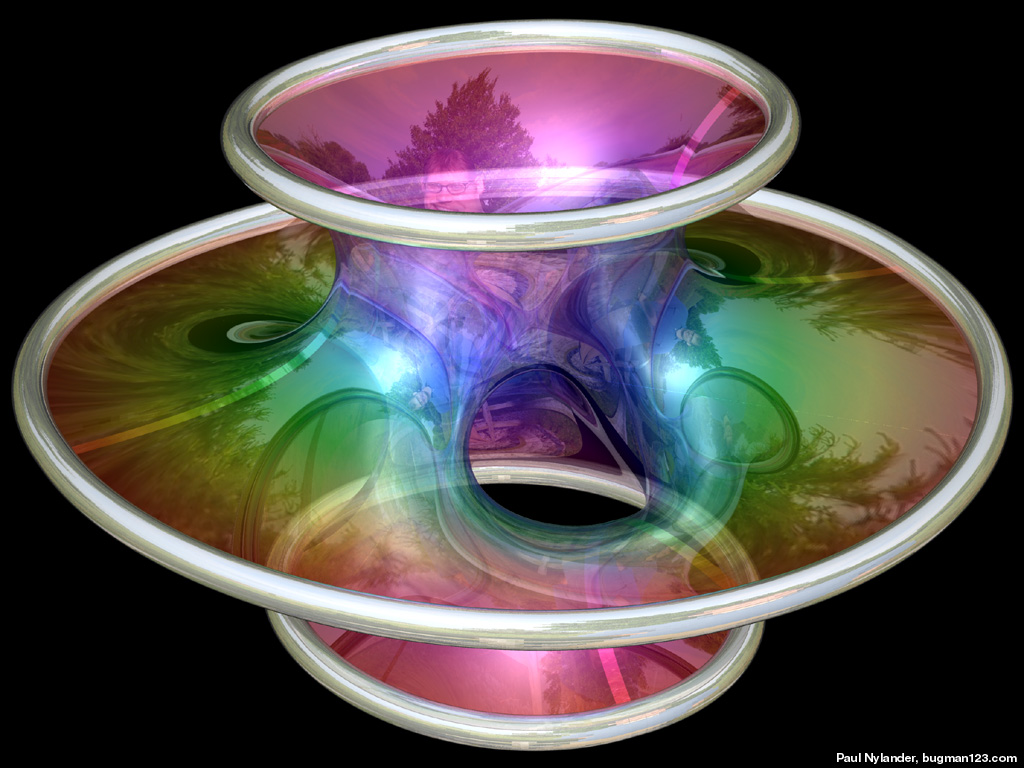# Elemente der Variationsrechnung

The calculus of variations is the study of (real-valued) functions of functions known as functionals, and in particular the search for minima and maxima of these functionals, so-called extremal functions. Many classical problems in mathematics and physics can be solved using the calculus of variations, by exploiting the fact that a solution of the problem, typically given by a differential equation, must be a minimiser or maximiser of a functional. Examples include the principle of least action, the theory of minimal surfaces (such as the description of soap bubbles), the problem of determining the shortest path between two points on a surface or manifold (geodesics), or brachistochrone curves (curves of fastest descent). Historically, the calculus of variations accompanied the development of other areas of analysis such as functional analysis and the theory of partial differential equations. It continues to find use as an important tool in these areas of mathematics, as well as in differential geometry (Morse theory), control theory, and of course in physics.
This is a short introductory bachelor level course in the methods and principles of the calculus of variations: how to derive a functional from a differential equation and vice versa, illustrated by various examples such as those listed above; typical methods for showing that an extremal function exists; and certain properties of these extrema. Along the way, we will give a brief introduction to Sobolev spaces, spaces of weakly differentiable functions which arise naturally in the search for solutions.

Prerequisite for the course is a solid grounding in analysis, such as that provided by Analysis 1 and 2 at Ulm University.

The course consists of one lecture per week and one tutorial every second week (2+1 SWS). It can be given in English or German, depending on the participants' preferences.Costas Minimalfläche [http://www.bugman123.com/MinimalSurfaces/Costa-large.jpg]

# Organisatorisches

## Termine und Räume

Vorlesung: Di 14-16 He22E18

Übung: Mo 14-16 N24/104 (2h zweiwöchig)

## Betreuung:

Dozent: James Kennedy
Übungsleiter: Adrian Spener

# Prerequisites and Exam

Prerequisite for the course is a solid grounding in analysis, such as that provided by Analysis 1 and 2 at Ulm University. The course is suitable for bachelor students in mathematics, economathematics and mathematical biometry.

The course consists of one lecture per week and one tutorial every second week (2+1 SWS, 4 ECTS). It can be given in English or German, depending on the participants' preferences.

The exam will be oral.

To take this course you need to register at Moodle.

# Literature

• Bernard Darocogna, Introduction to the calculus of variations, 3rd edition, Imperial College Press, London, 2015.
• Lawrence C. Evans, Partial di fferential equations, 2nd edition, American Mathematical Society, Providence, R.I., 2010.
• Haim Brezis, Functional Analysis, Sobolev Spaces and PDEs.
• Giuseppe Buttazzo, Mariano Giaquinta, and Stefan Hildebrandt, One dimensional variational problems.
• Bernard Dacorogna, Direct methods in the calculus of variations.
• Mariano Giaquinta and Stefan Hildebrandt, Calculus of variations I.
• Enrico Giusti, Direct Methods in the Calculus of Variation.